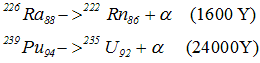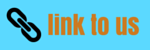# Unit of activity|Alpha Decay

### 4) Unit of activity

• The most commonly used unit is the curie
• Curie was originally based on the rate of decay of a gram of radium
• There are $3.7 \times 10^{10}$ disintegrations per sec per gram of radium .This no is taken as a standard
=> One curie=$3.7 \times 10^{10}$ disintegrations per sec
• One curie of activity is very strong source of radiation
=> 1 milli curie=1mCi=$10^{-3}$Ci
1 microcurie=1μCi=$10^{-6}$ Ci
• Another unit of activity is Rutherford
$1rd=10^6$ dis/sec
• Activity |dN/dt|=λN=.693N/T
=> A very short lived substance gives rise to large activity ,even it is present in minute quantities
• The SI unit of radioactivity recently proposed is Becquerel (Bq) which is defined as activity done to one disintegration per sec hence
1ci=$3.7 \times 10^{10}$ bq
=37G bq

### 5) Alpha decay:

• Nucleus before the decay is called parent nucleus and after the decay is called daughter nucleus
• In Alpha decay, the parent nucleus AXZ emits an α particle (=4He2) leaving behind a daughter nucleus of four mass unit less and two charge units less i.e. A-4XZ-2• α decay shift the element two places to the left in the periodic tables of elements ex• All nuclides of A >= 210 and Z > 83 tends to decay by α emission
• 209Bi is the heaviest stable nuclide in nature
• α decay in heavy nucleus occur because a too heavy nucleus becomes unstable due to coulomb repulsion and by emitting an α particle the nucleus decrease its A and Z to moves towards stability
• Now the rest mass energy of parent nucleus AXZ is greater then the sum of rest mass energies of A-4XZ-2 and 4He2
• The difference between the rest mass energies of initial constituents and final products is called Q-value of the process
• For α decay process ,Q value is
Q=[mp -(md+mα)]c2
where mp -> Mass of parent nucleus ZAX
md -> Mass of parent nucleus Z-2A-4X
mα -> Mass of parent nucleus 24He